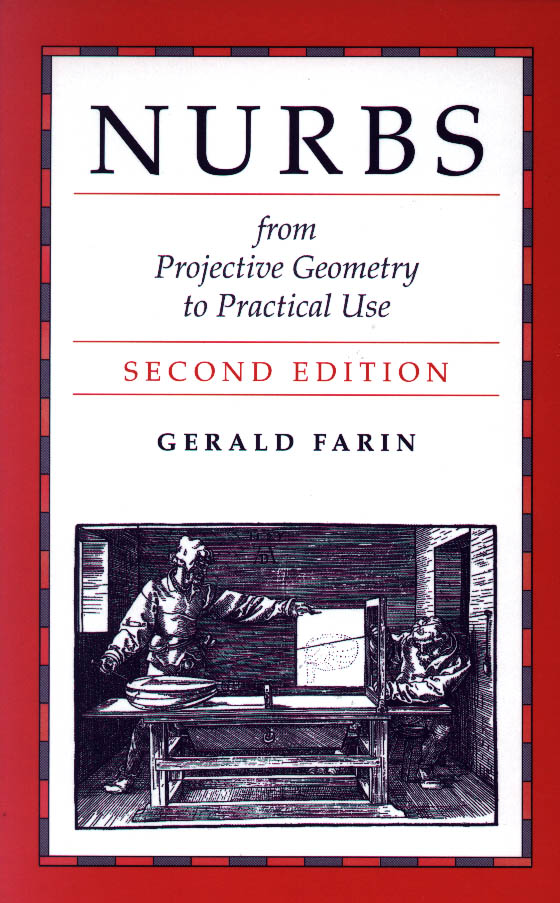# NURBS for Curve and Surface Design from Projective Geometry to Practical Use 2nd edition

by Gerald Farin

Published in 1999 by AK Peters, Ltd.
267 Pages
ISBN 1-56881-084-9

### 1. The Projective Plane

Motivation, The projective plane, Points and lines, Projective line coordinates, Projective plane coordinates, Ranges and pencils, Pappus' theorem, Menelaos' Theorem, Duality, The extended affine plane, Topological aspects, Parametric lines, Lines and line segments, Projective three-space, Problems,

### 2. Projective Maps

Perspectivities, Cross ratios, Projectivities, Moebius transformations, Collineations, Constructing Collineations, Affine maps, Problems,

### 3. Conics

Line and point conics, Conic constructions, Points and lines, Pascal's theorem, Affine conics, Problems,

### 4. Conics in Parametric Form

Parametric curves in P2, The Bernstein form of a conic, The Dual Form, Derivatives, The implicit form, Implicit interpolating conics, Parametric interpolating conics, Blossoms and polars, Reparametrization, The complementary segment, Parametric affine conics, Problems

The rational quadratic form, Conic classification, Properties of rational quadratics, Derivatives, Control vectors, The parabola, The circle, Problems,

### 6. Conic splines

C1 conditions, Parametric continuity for rational conic splines, Conic B-splines, Curvature continuity, G2 Conic splines, Interpolation with G2 conic splines, Higher order smoothness, Problems

### 7. Rational Bezier curves

The Bernstein form, The de Casteljau algorithm, Degree elevation, Degree reduction, Reparametrization, Nonparametric curves, Base points, Derivatives, Smoothness, Blossoms, Control vectors, Duality, Hybrid Bezier curves, Straight lines as Bezier curves, Problems

### 8. Rational Cubics

Rational cubic conics, Embedding cones, The embedding bilinear patch, Asymptotes, The Hermite form, Cubic Lagrange interpolation, Osculatory interpolation, Problems

### 9. Pythagorean Curves

Circles and the stereographic projection, Pythagorean hodograph curves, Rational PH offsets, Rational offsets, Rational curves on the sphere, Problems

### 10. Rectangular Patches

Bilinear patches, Bezier patches, De Casteljau algorithms and blossoms, The weight points, Derivatives and continuity, Reparametrization, Rectangular NURBS surfaces, Blossoms, Surfaces of revolution, Developable surfaces, Problems

### 11. Rational Bezier Triangles

Projective Bezier triangles, Degree elevation, Blossoms and derivatives, Barycentric coordinates, Rational Bezier triangles, Problems